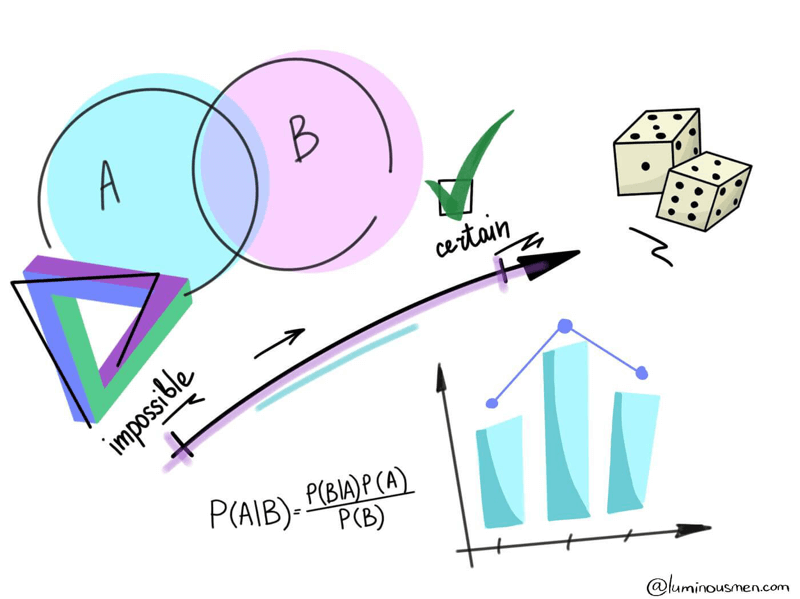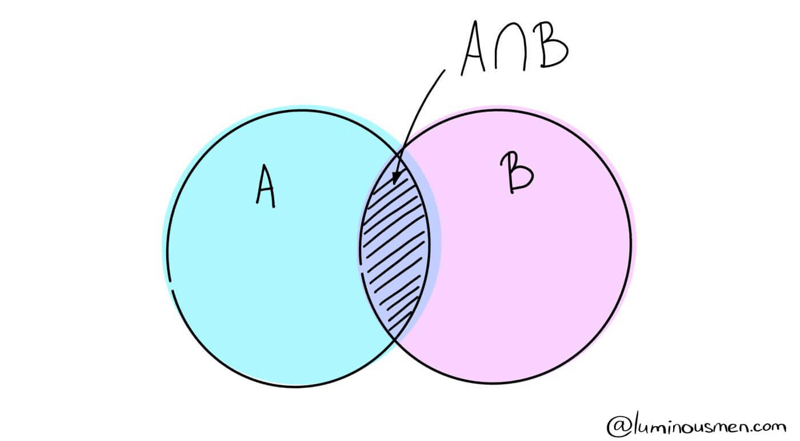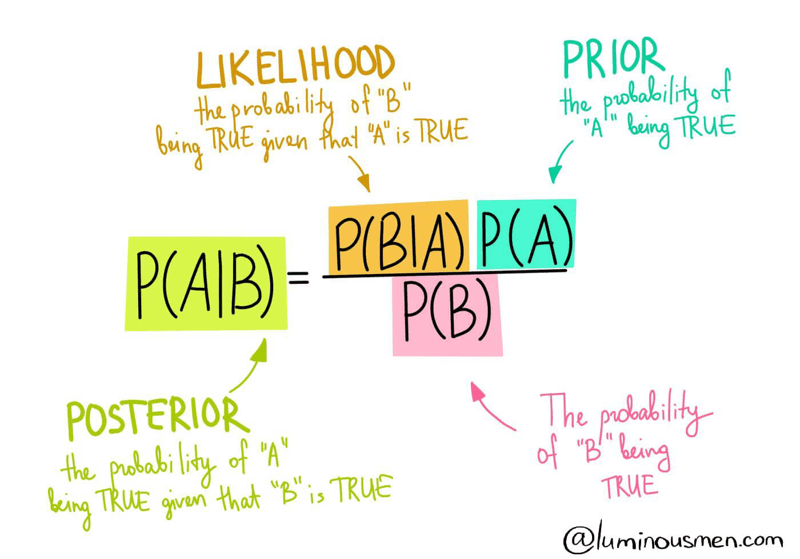# Data Science. Bayes theoremThere are a lot of engineers who have never been involved in the field of statistics or data science. But in order to build a data science pipelines or rewrite produced code by data scientists to an adequate, easily maintained code many nuances and misunderstandings arise from the engineering side. For those Data/ML engineers and novice data scientists, I make this series of posts. I'll try to explain some basic approaches in plain English and, based on it, explain some of the Data Science basic concepts.

The whole series:

Bayes theorem is one of the most important concepts of probability theory used in Data Science. It allows us to update our beliefs based on the appearance of new events.

## Intuitive understanding

The man was sitting with his back to a perfectly flat and perfectly square table. Then he asked his assistant to throw the ball on the table. Obviously, this ball could have landed and ended up anywhere on the table. The man wanted to find out where it is ended up but in a more analytical way.

So, he asked his assistant to throw another ball and tell him if it landed on the left or on the right, or on the front or at the back of the first ball. He wrote that down, and then he asked him to throw more and more balls on the table. He understood that with this method he could update his idea of where the first ball was landed**. But of course, he could never be completely sure, but with each new proof, he would become more and more accurate.

And that's how Beyes saw the world, that's his thinking experiment. It's not that he thought that the world is not defined, that reality doesn't exist, but that we can't know it perfectly, and all we can hope to do is to renew our understanding as more and more evidence emerges.

## Practical explanation

Imagine we have two related events A and B. It can be, for example, A — I get wet today, B — it will be rainy today. In one way or another, many events are related to each other, as in our example. Let's calculate the probability of A given B has already happened.Now since B has happened, the part which now matters for A is the shaded part which is interestingly A ∩ B. So, the probability of A given B turns out to be:

$$P(A|B) = \frac{P(A ∩ B)}{P(B + A ∩ B)}$$

Therefore, we can write the formula for event B given A has already occurred by:

$$P(A|B) = \frac{P(A ∩ B)}{P(B)}$$

or

$$P(B|A) = \frac{P(A ∩ B)}{P(A)}$$

Now, the second equation can be rewritten as :

$$P(A|B) = \frac{P(B|A)P(A)}{P(B)}$$

That's all. That's all the conclusions that have to be drawn to come to the Bases theorem. Let's combine it all into a single picture and rename the members of the formula:• P(A|B) is the posterior probability or the probability of A to occur given event B already occurred
• P(B|A) is the likelihood, or the probability of B given A
• P(A), P(B) is the prior probability of event A and B to occur

It should be noted that with independent events P(B | A) = P(B), which is logical — if the occurrence of event A does not affect the occurrence of event B.

Example: If a single card is drawn from a standard deck of playing cards, the probability that the card is a king is 4/52, since there are 4 kings in a standard deck of 52 cards. Paraphrasing this, if a king is an event "this card is a king," the prior probability P(King) = 4/52.

If evidence is provided (for instance, someone looks at the card) that the single card is a face card, then the posterior probability P(King|Face) can be calculated using Bayes theorem formula:

P(King|Face) = P(Face|King) * P(King) / P(Face)

Since every King is also a face card, P(Face|King) = 1. Since there are 3 face cards in each suit (Jack, Queen, King), the probability of a face card is P(Face) = 12/52.

Using Bayes' formula gives P(King|Face) = 1 * 4/52 / 12/52 = 4/12 = 1/3.

## Try it yourselfBuy me a coffee